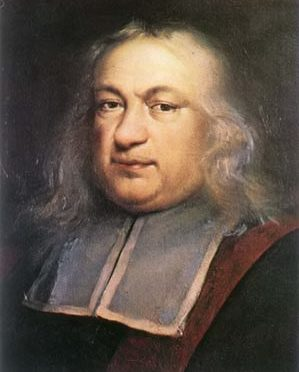# Tag Archives: Fermat's last theorem# Basque Fact of the Week: Was the Mathematician Pierre de Fermat Basque?

Pierre de Fermat was one of the most recognized mathematicians of his time, and perhaps in all of history. He was infamous for making claims of mathematical proofs in the margins of documents without actually giving the proof. Ever since, mathematicians have struggled to prove his theorems. His Last Theorem, which relates to number theory, […]